Courses

# Test: Previous Year Questions: Thermodynamics

## 30 Questions MCQ Test Chemistry Class 11 | Test: Previous Year Questions: Thermodynamics

Description
This mock test of Test: Previous Year Questions: Thermodynamics for JEE helps you for every JEE entrance exam. This contains 30 Multiple Choice Questions for JEE Test: Previous Year Questions: Thermodynamics (mcq) to study with solutions a complete question bank. The solved questions answers in this Test: Previous Year Questions: Thermodynamics quiz give you a good mix of easy questions and tough questions. JEE students definitely take this Test: Previous Year Questions: Thermodynamics exercise for a better result in the exam. You can find other Test: Previous Year Questions: Thermodynamics extra questions, long questions & short questions for JEE on EduRev as well by searching above.
QUESTION: 1

### The quantity required to increase the temperature of a body by 1 Kelvin is called [AIEEE-2002]

Solution:

Thermal Capacity

The amount of heat required to raise the temperature of a body through 1K is called the heat capacity of that body. The heat capacity depends on the material and the mass of the body. Its unit is JK-1. The heat capacity of a body is 10 JK-1 means 10 J heat is required to raise the temperature of the body through 1K.

Let, Q amount heat is required to increase the temperature of a body through Δθ.

Therefore the amount of heat required to raise tilde temperature of the body through 1K is Q/ Δθ Heat capacity, C = Q / Δθ

QUESTION: 2

### Even Carnot engine cannot give 100% efficiency because we cannot –  [AIEEE-2002]

Solution:

Efficiency of a carnot engine is given by

η = 1 - Q2/Q1

In order to achieve 100% efficiency (η=1), Q2 must be equal to 0 which means that all the heat form the source is converted to work.

The temperature of sink means a negative temperature on the absolute scale at which the temperature is greater than unity. This would be violation of second law of thermodynamics. Hence negative temperature of absolute scale is impossible and we cannot reach absolute 0 temperature.

QUESTION: 3

### Which statement is incorrect ?  [AIEEE-2002]

Solution:

Out of given statements :
We know that carnot cycle is reversible cycle, and reversible cycle has maximum efficiency of all other cycles and so only statement A is incorrect.

QUESTION: 4

"Heat cannot be itself flow from a body at lower temperature to a body at higher temperature" is a statement or consequence of –

[AIEEE - 2003]

Solution:

Heat cannot flow itself from a lower temperature to a body of higher temperature (for cyclic process this is Kelvin-Planck statement). This corresponds to second law of thermodynamics.

QUESTION: 5

Which of the following parameters does not characterize the thermodynamic state of matter ?

[AIEEE-2003]

Solution:

The work done does not characterize a thermodynamic state of matter. It gives only a specific relationship (depending on path ) between two different thermodynamic state.

QUESTION: 6

A Carnot engine takes 3 × 106 cal. of heat from a reservoir at 627ºC, and gives it to a sink at 27º C. The work done by the engine is –

[AIEEE-2003]

Solution: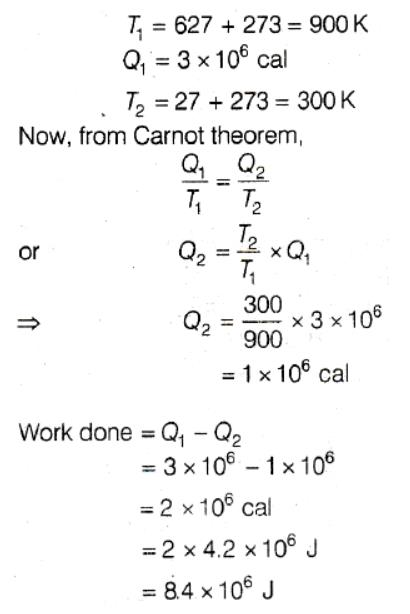QUESTION: 7

In an irreversible process taking place at constant T and P and in which only pressure-volume work is being done the change in Gibbs free energy (dG) and change in entropy (dS) satisfy the criteria -

[AIEEE-2003]

Solution:

For spontaneous process, dS > 0 and dG should be negative i.e. < 0.

QUESTION: 8

The internal energy change when a system goes from state A to B is 40 kJ/mole. If the system goes from A to B by a reversible path and returns to state A by an irreversible path what would be the net change in internal energy

[AIEEE-2003]

Solution:

For a cyclic process, the net change in internal energy is equal to zero and change in the internal energy does not depend on the path by which the final state is reached.

QUESTION: 9

The correct relationship between free energy change in a reaction and the corresponding equilibrium constant KC is –

[AIEEE-2003]

Solution:

∆G = ∆G° + RTlnK
In equilibrium, K = Kc, so ∆G =0
So, 0 = ∆G° +RTlnKc
-∆G° =RTlnKc

QUESTION: 10

Which of the following statements is correct for any thermodynamic system ?

[AIEEE-2004]

Solution:

Internal energy does not change in isothermal process. ΔS can be zero for adiabatic process. Work done in adiabatic process may be non-zero.

QUESTION: 11

An ideal gas expands in volume from 1 × 10-3 m3 to 1 × 10-2 m3 at 300 K against a constant pressure of 1 × 105 Nm-2. The work done is -

[AIEEE-2004]

Solution:

The correct answer is option A
Work done at constant temperature is
W = −PΔV
W = − (P) x (V2​−V1​)
W = − (1 x 105) x (10−2 - 10−3)
W = − 900J

QUESTION: 12

Which of the following is incorrect regarding the first law of thermodynamics ?

[AIEEE-2005]

Solution:
QUESTION: 13

The temperature-entropy diagram of a reversible engine cycle is given in the figure. Its efficiency is -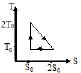[AIEEE-2005]

Solution:

From the graph, we can say that area under curve = work done = ½ ×So×To
And QBC = Area under line BC.
Area Under BC = ½×So×3To = 3ToSo/2
η = ∆W/QBC = (SoTo/2)/(3SoTo/2) = 1/3

QUESTION: 14

A system goes from A to B via two processes I and II as shown in figure. If ΔU1 and ΔU2 are the changes in internal energies in the processes I and II respectively, then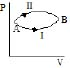[AIEEE-2005]

Solution:

As internal energy is state function or independent of path. So it only depends upon initial and final position. By this way, option a is correct.

QUESTION: 15

Consider the reaction : N2 + 3 H2 → 2 NH3 carried out at constant temperature and pressure. If ΔH and ΔU are the enthalpy and internal energy changes for the reaction, which of the following expressions is true ?

[AIEEE-2005]

Solution:

∆H = ∆U-∆ngRT,
where ∆ng = ∑Molesgaseous product - ∑Molesgaseous reactant
Here, ∆ng = 2-4 =2 = -ve.
So ∆H < ∆U

QUESTION: 16

The work of 146 kJ is performed in order to compress one kilo mole of a gas adiabatically and in this process the temperature of the gas increases by 7º C. The gas is - (R = 8.3 J mol-1 K-1) –

[AIEEE 2006]

Solution:

For adiabatic compression, ∆Q = 0
Using 1st law of thermodynamics,
∆U = -W
nCv∆T = -W
nf/2 R∆T = -W
f = -2W/nR∆T =  -2×-146/1×8.3×7
f ∼ 5
Therefore, degree of freedom = and so the gas is diatomic.

QUESTION: 17

An ideal gas is allowed to expand both reversibly and irreversibly in an isolated system. If Ti is the initial temperature and Tf is the final temperature, which of the following statements is correct

[AIEEE 2006]

Solution:

The correct answer is option D
According to first law of thermodynamics
ΔQ =ΔU + ΔW
An isolated system is adiabatic. This means ΔQ = 0.
The first law in this case yields
0= ΔU + ΔW ⇒ ΔW=−ΔU… (1)
For expansion, ΔW is positive and hence ΔU is negative. This means Tf is less than Ti in both the cases. However it is interesting to see in which case the final temperature is greater.
For the same expansion of volume, the work done in an irreversible process is greater than that in a reversible one because the system has to work against friction etc. Thus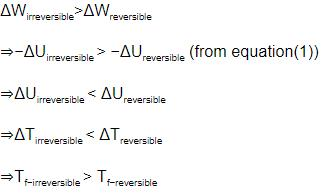QUESTION: 18

(ΔH - ΔH) for the formation of carbon monoxide (CO) from its elements at 298 K is (R = 8.314 J K-1 mol-1)–

[AIEEE 2006]

Solution: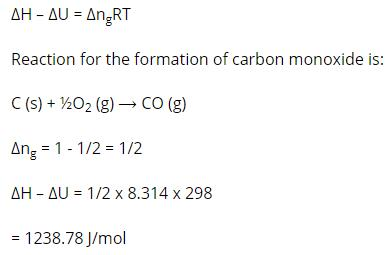QUESTION: 19

A Carnot engine, having an efficiency of h = 1/10 as heat engine, is used as a refrigerator. If the work done on the system is 10 J, the amount of energy absorbed from the reservoir at lower temperature is

[AIEEE 2007]

Solution:

β = (1-η)/η
= (1-1/10)/(1/10) = (9/10)/(1/10) = 9
β = Q2/W
Q = W × β = 10 × 9 = 90J

QUESTION: 20

When a system is taken from state i to state f along the path iaf, it is found that Q = 50 cal and W = 20 cal. Along the path ibf Q= 36 cal. W along the path ibf is -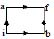[AIEEE 2007]

Solution:

From 1st law of Thermodynamics,
∆Q = ∆U + W
For path iaf,
50 = ∆U + 20
∆U = 30 cal
For path ibf,
∆Q = ∆U + W
W = ∆q - ∆U
= 36 - 30 = 6 cal

QUESTION: 21

In conversion of lime-stone to lime, CaCO3(s) → CaO(s) + CO2(g) the values of ΔHº and ΔSº are + 179.1 kJ mol-1 and 160.2 J/K respectively at 298K and 1 bar. Assuming that ΔHº and ΔSº do not change with temperature, temperature above which conversion of limestone to lime will be spontaneous is-

[ AIEEE 2007]

Solution:

We know, ∆G = ∆H-T∆S

So, lets find the equilibrium temperature, i.e. at which

∆G = 0

∆H-T∆S

T= 179.1 x 1000/160.2

= 1118 K

So, at the temperature above this, the reaction becomes will spontaneous.

QUESTION: 22

Assuming that water vapour is an ideal gas, the internal energy change(ΔU) when 1 mol of water is vapourised at 1 bar pressure and 100°C, (Given : Molar enthalpy of vapourisation of water at 1 bar and 373 K = 41 kJ mol-1 and R = 8.3 J mol-1K-1 ) will be -

[AIEEE 2007]

Solution:

H2O(l) → H2O(g)
∆ng = 1 - 0 = 1
∆H = ∆U + ∆ngRT
∆U = ∆H - ∆ngRT
= 41-8.314×10-3×373
= 37.9 kJ mol-1

QUESTION: 23

Identify the correct statement regarding a spontaneous process –

[AIEEE 2007]

Solution:

Spontaneity of reaction depends on tendency to acquire minimum energy state and maximum randomness. For a spontaneous process in an isolated system, the change in entropy is positive.

QUESTION: 24

Standard entropy of X2, Y2 and XY3 are 60, 40 and 50 J K-1 mol-1, respectively. For the reaction,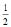X2 +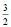Y2 – XY3 DH = – 30 kJ, to be at equilibrium, the temperature will b[AIEEE 2008]

Solution: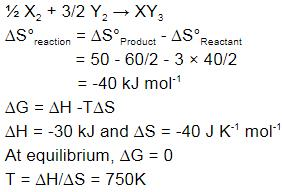QUESTION: 25

In a fuel cell methanol is used as fuel and oxygen gas is used as an oxidizer. The reaction is CH3OH(l) + O2(g) → CO2(g) + 2H2O(l) At 298 K standard Gibb's energies of formation for CH3OH(l), H2O(l) and CO2(g) are –166.2, –237.2 and –394.4 kJ mol-1 respectively. If standard enthalpy of combustion of methanol is –726 kJ mol-1, efficiency of the fuel cell will be-

[AIEEE 2009]

Solution: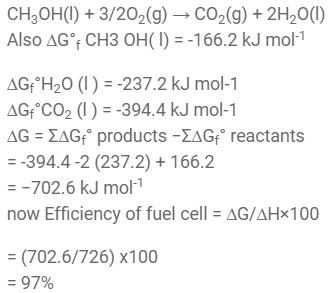QUESTION: 26

A diatomic ideal gas is used in a Carnot engine as the working substance. If during the adiabatic expansion part of the cycle the volume of the gas increases from V to 32 V, the efficiency of the engine is-

Solution: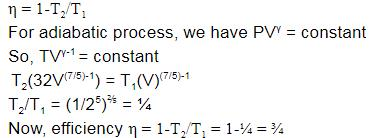QUESTION: 27

For a particular reversible reaction at temperature T, ΔH and ΔS were found to be both +ve. If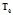is the temperature at equilibrium, the reaction would be spontaneous when

[AIEEE 2010]

Solution:

For a particular reversible reaction at T temperature

ΔG = ΔH-TΔS

When ΔH, S is positive

ΔG = +ΔH- T (+ΔS)

For a spontaneous process, ΔG must be negative, it is possible only at high temperature.

That mean T> Te

QUESTION: 28

The entropy change involved in the isothermal reversible expansion of 2 moles of an ideal gas from a volume of 10 dm3 to a volume of 100 dm3 at 27º C is :

[AIEEE 2011]

Solution:

∆S = nRT ln(v2/v1)
= 2.30328.314 log(100/10)
= 38.3 J mol-1 K-1

QUESTION: 29

In view of the signs of DrG° for the following reactions :

PbO2 + Pb → 2 PbO, DrG° < 0

SnO2 + Sn → 2 SnO, DrG° > 0,

which oxidation states are more characteristic for lead and tin ?       [AIEEE 2011]

Solution:

The correct answer is option C
PbO2​​ + Pb​ →​ 2PbO      ​ΔG >0Spontaneous +2 is more stable
+4       0         + 2
SnO2 ​+ Sn​→​2SnO      ​ΔG>0NonSpontaneous + 2 less stable
​   +4       0       +2
So for lead +2 and for Sn +4

QUESTION: 30

The incorrect expression among the following is –

[AIEEE-2012]

Solution:

The correct answer is Option B.

ΔGo = −RTlnK.........(i)
ΔGo = ΔHo − TΔSo......(ii)
From (i) and (ii),
−(ΔHo + TΔSo) = RT lnK